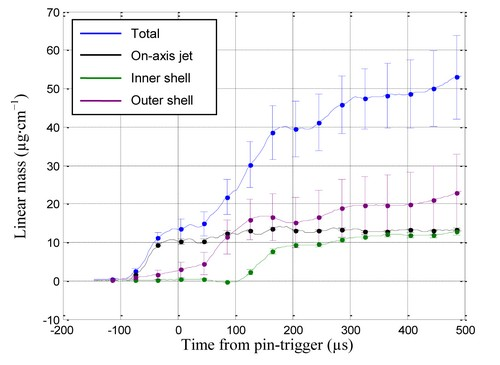Plasma Laboratory - Weizmann Institute of Science

## Z-pinch 1D interferometry results

Since each measurement provides information of the local region covered by the probe beam, numerous measurements are needed to obtain the density distribution for each axial location. The spatial resolution and accuracy are determined by the probe beam diameter and by the number of measurements taken at each point.

The method of interferometry cannot be used to measure the density distribution of the gas-puff directly, since the fringe shift picked up by the photodiode is determined by a line integral over the density in the chord the beam traverses. However, if the gas-puff can be assumed to be axisymmetric Abel inversion of the data gives the radial density distribution. This distribution can then be compared with normalized density distributions obtained from PLIF measurements, and serve to calibrate those measurements.Fig. ‎1: Radial density distribution of a triple gas-puff at t = 404 µs with respect to the pin‑trigger
obtained from interferometry, compared with a scaled ICCD PLIF intensity profile at z = 2 mm.

Since the interferometric measurements are obtained as a function of time, integration over the density inferred using Abel inversion yields the linear mass as a function of time. Fig. 2 shows such data for each puff of the triple valve-nozzle system, as well as the total linear mass.Fig. ‎2: Linear mass of the triple gas-puff as a function of time.

^ Top
Modified on: 2010-05-23